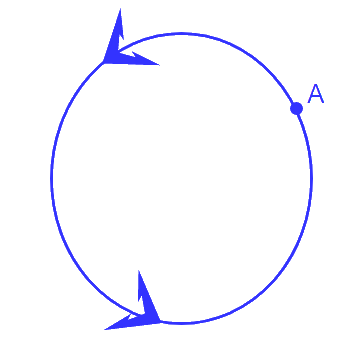# Ex.1.2 Q7 Real Numbers Solution - NCERT Maths Class 10

There is a circular path around a sport field. Sonia takes $$18$$ minutes to drive one round of the field, while Ravi takes $$12$$ minutes for the same. Suppose they both start at the same point and at the same time and go in the same direction. After how many minutes will they meet again at the starting point?{%--answer--%}

What is known?

• Sonia takes $$18$$ minutes to drive one round of the field.
• Ravi takes $$12$$ minutes for the same.
• They both start at the same point and at the same time and go in the same direction.

What is unknown?

After how many minutes will they meet again at the same point.

Reasoning:

Time taken by Sonia is more than Ravi to complete one round. Now, you have to find after how many minutes will they meet again at the same point. For this, there will be a number which is a divisible by both $$18$$ and $$12$$ and that will be the time when both meet again at the starting point. To find this you have to take LCM of both the numbers.

Steps:

LCM of $$18$$ and $$12$$,

\begin{align}18 &= 2 \times 3 \times 3\\12 &= 2 \times 2 \times 3\end{align}

LCM of $$12$$ and $$18$$

\begin{align}&= 2 \times 2 \times 3 \times 3\\ &= 36\end{align}

Therefore, Ravi and Sonia will meet together at starting point after $$36$$ minutes.

Download SOLVED Practice Questions of Ex.1.2 Q7 Real Numbers Solution - NCERT Maths Class 10 for FREE
Ex 1.2 | Question 7
Instant doubt clearing with Cuemath Advanced Math Program